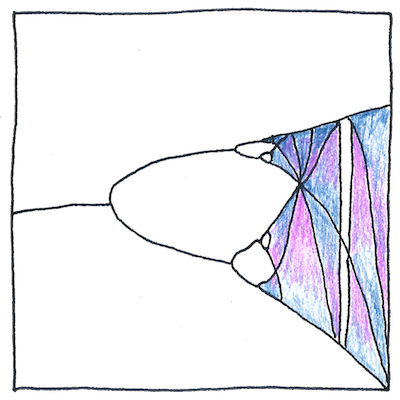# 1903

## The book of science

Tom Sharp

 Henri Poincaré mathematics

## Chaos theory

• Small changes to even a simple solar system
• governed by Isaac Newton’s laws
• cause intricate and unpredictable effects,
• according to an analysis by Henri
• Poincaré, the French mathematician.
• *
• Henri Poincaré, the mathematician, realized
• that minor differences in initial conditions
• can result in major differences in the universe
• where planets could run around like ants.

## The three-body problem

• Poincaré tried but
• did not solve the
• three-body problem
• —where three planets
• are subject to only their
• own gravitational attractions
• —because he found
• even when everything
• was deterministic and
• nothing was random
• that such a system
• was unpredictable.

## Indeterminism

• Many disciplines
• lack discipline.
• Too often, the cost
• of explaining would exceed
• the value of the explanation.

A dynamic system is chaotic if it is sensitive to initial conditions, if each element doesn’t stay out of the others’ way, and if its orbits eventually fill the space. It is nice to be precise; however, the term chaos precedes the science and means formless or completely disordered.